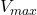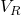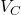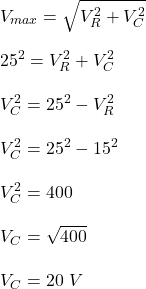## An RC series circuit is connected to an ac generator with a maximum emf of 25 V. If the maximum potential difference across the resistor is

Question

An RC series circuit is connected to an ac generator with a maximum emf of 25 V. If the maximum potential difference across the resistor is 15 V, then the maximum potential difference across the capacitor is

in progress 0
5 months 2021-08-18T11:32:03+00:00 1 Answers 0 views 0

The maximum potential difference across capacitor is 20 V

Explanation:

Given;

maximum emf of the ac circuit,= 25 V

maximum potential difference across resistor,= 15 V

maximum potential difference across capacitor,= ?

Determine the maximum potential difference across capacitor using the equation below;Therefore, the maximum potential difference across capacitor is 20 V